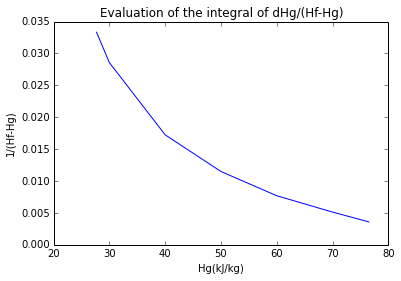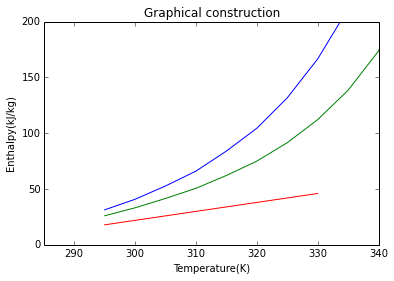# Chapter 13:Humidification and water cooling¶

## Example no:13.1,Page no:740¶

In :
#Variable declaration
P=101.3e3
T=297
R=8314  #gas constant
RH=60  #Relative humidity
p_b1=12.2e3 #Vapor pressure at 297 K
p_b2=6e3  #Vapor pressure at 283 K
M_w=78  #molecular weight of benzene
M_a=28  #Mass of nitrogen

#Calculation
#From the definition of percentage relative humidity (RH)
P_w=(p_b1)*(RH/100.0)
#In the benzene -nitrogen mixture:
m_b=P_w*M_w/(R*T) #mass of benzene
m_n=(P-P_w)*M_a/(R*T) #mass of nitrogen
H=m_b/m_n  #Humidity at 297 K
#In order to recover 80 per cent of the benzene, the humidity must be reduced to 20 per cent of the initial value
H_o=H*.20
#Thus in equation 13.2
P_r=p_b2+(p_b2/M_a*M_w)/H_o

#Result
print"\n The required pressure is =",round(P_r*1e-3,1),"kN/m**2"

 The required pressure is = 391.2 kN/m**2


## Example no:13.2,Page no:741¶

In :
#Variable declaration
P=101.3e3  #Given pressure
T=300  #Given Temperature
RH=25  #Percentage relative humidity of water
P_wo=3.6e3  #partial pressure of water vapour when air is saturated with vapour
M_w=18  #Molecular weight of water
M_a=29  #Molecular weight of air
R=8314  #gas constant

#Calculation
P_w=P_wo*(RH/100.0)
m_w=P_w*M_w/(R*T) #mass of water vapour
m_a=(P-P_w)*M_a/(R*T) #mass of water air
Vs_w=1/m_w #specific volume of water vapour at 0.9 kN/m**2
Vs_a=1/m_a #specific volume of air at 100.4 kN/m**2
H=m_w/m_a #Humidity
H_v=Vs_a #Humid volume
H_p=(P-P_wo)/(P-P_w)*RH  #Percentage humidity

#Result
print"(a) The partial pressure of the water vapour in the vessel = ",P_w*1e-3,"kN/m**2"
print" b) Specific volume of water vapour =",round(Vs_w),"m**3/kg"
print"    Specific volume of air =",round(Vs_a,3),"m**3/kg"
print"(c) Humidity of air =",round(H,4),"kg water/kg air"
print"    Humid volume =",round(H_v,3),"m**3/kg"
print"(d) Percentage humidity =",round(H_p,1),"per cent"

(a) The partial pressure of the water vapour in the vessel =  0.9 kN/m**2
b) Specific volume of water vapour = 154.0 m**3/kg
Specific volume of air = 0.857 m**3/kg
(c) Humidity of air = 0.0056 kg water/kg air
Humid volume = 0.857 m**3/kg
(d) Percentage humidity = 24.3 per cent


## Example no:13.3,Page no:743¶

In :
#Variable declaration
T=310.0  #Temperature of moist air
T_w=300.0  #Wet bulb tempeature
L=2440e3  #Latent heat of vapourisation of water at 300 K
P=105e3  #Given total pressure
P_wo1=3.6e3  #Vapour pressure of water vapour at 300 K
P_wo2=6.33e3  #Vapour pressure of water vapour at 310 K
M_w=18.0  #Molecular weight of water
M_a=29.0  #Molecular weight of air

#Calculation
import sympy
H_w=(P_wo1/(P-P_wo1))*(M_w/M_a)  #The humidity of air saturated at the wet-bulb temperature
#Therefore, taking (h/hD*rho*A) as 1.0 kJ/kg K, in equation 13.8:
H=H_w-(1e3/L)*(T-T_w)
#In equation 13.2:
x=sympy.Symbol('x')
P_w=sympy.solve(H*(P-x)*M_a-M_w*x)
RH=P_w/P_wo2*100.0

#Result
print"\n The humidity of the air =",round(H,3),"kg/kg"
print"\n The percentage relative humidity (RH)=",round(RH,1),"per cent"

 The humidity of the air = 0.018 kg/kg

The percentage relative humidity (RH)= 46.6 per cent


## Example no:13.4,Page no:749¶

In :
#Variable declaration
#Refer HUMIDITY ENTHALPY PLOT Figure 13.5 Page 748 as Humidity Chart
#According the given passes and situatuion
T = [325,301,308,312,315]        #[K]
H = [.005,.015,.022,.027,.032]    #[kg/kg]
#From Humidity Chart on humidifying to 60 percent humidity
Tw = [296,301,305,307]            #[K]

#Calculation
Hin = H-H            #[kg/kg] Increase in Humidity
#From Humitidy Chart at the obtained leaving conditions
v = .893         #[m**3/kg] Specific Volume of dry air
vs = .968        #[m**3/kg] Specific Volume of Saturated air
vh = .937        #[m**3/kg] Humid Volume of air of 60 per cent humidity by Interpolation of Curve in Humidity Chart
x = 5        #[m**3/s] Amount of moist air leaves the dryer in (b)
m = x/vh        #[kg/s] Mass of air passing through the dryer
mw = m*Hin      #  [kg/s] Mass of water evaporated
Tb = 370.0            #[K] dry Bulb temperature corresponding to humidity of .005 kg/kg and wet-bulb temperature 307 K

#Result
print'\n\n (a) The temperature of the material on each tray (in Kelvin)'
print "\t",Tw,"K"
print' Thus the air leaving the system is at',T,' K and 60 per cent humidity.'
print'\n\n (b) If 5 m**3/s moist air leaves the dryer, The amount of water removed is',round(mw,3),' kg/s.'
print'\n\n (c) The Temperature to which the inlet air would have to be raised\n     to carry out the drying in single stage is',Tb,'K.'


(a) The temperature of the material on each tray (in Kelvin)
[296, 301, 305, 307] K
Thus the air leaving the system is at 315  K and 60 per cent humidity.

(b) If 5 m**3/s moist air leaves the dryer, The amount of water removed is 0.144  kg/s.

(c) The Temperature to which the inlet air would have to be raised
to carry out the drying in single stage is 370.0 K.


## Example no:13.5,Page no: 753¶

In :
#Variable declaration
G1=1  #flow rate of air at 350 K
PH1=10  #Percentage Humidity at 350 K
G2=5  #flow rate of air at 300 K
PH2=30  #Percentage Humidity at 300 K
#from fig 13.4
H1=0.043  #Humidity at 350 K and 10 percent humidity
H2=0.0065  #Humidity at 300 K and 30 percent humidity

#Calculation
import sympy
#Thus, in equation 13.23:
H=((G1*H1)+(G2*H2))/(G1+G2)
#from fig 13.5
H_1=192e3 #Entahlpy at 350 K and 10 percent humidity
H_2=42e3 #Enthalpy at 300 K and 30 percent humidity
x=sympy.Symbol('x')
H_=sympy.solve((G1*(x-H_1))-(G2*(H_2-x)))
#From Figure 13.5:
#at H_ (Enthalpy)= 67 kJ/kg and H(humidity) = 0.0125 kg/kg
T=309

#Result
print"\n Humidity of final stream =",round(H,4),"kg/kg"
print"\n Entahlpy of the resultant stream =",round(H_*1e-3),"kJ/kg"
print"\n Temperature of the resultant stream =",T,"K"

 Humidity of final stream = 0.0126 kg/kg

Entahlpy of the resultant stream = 67.0 kJ/kg

Temperature of the resultant stream = 309 K


## Example no:13.6,Page no:755¶

In :
#Variable declaration
G_s=0.15  #Mass flow rate of steam
T=400  #Temperature to which steam is superheated
T_a=320 #Tremperature of air
RH_a=20  #Percentage relative humidity of air
G_a=5 #Mass flow rate of air
L=2258e3  #latent heat of steam
Cp=2e3  #Specific heat of superheated steam

#Calculation
#Enthalpy of steam
H_3=4.18*(373-273)+L+Cp*(T-373)
#From Figure .13.5:
#at T=320 K and 20 percent Relative Humidity
H1=0.013 #Humidity
H_1=83e3 #Enthalpy
#By making required constructions we get
H=0.043
H_=165e3
T_s=324
#from chart and equation 13.28
G_case2=0.41

#Result
print"\n Relative humidity of stream=",round(H,3),"kg/kg"
print" Entahlpy of stream =",H_*1e-3," kJ/kg"
print" Temperature of stream =",T_s,"K"
print"\n\n When exit temperature = 330 K"
print" The required flow of steam = ",round(G_case2,2)," kg/s"

 Relative humidity of stream= 0.043 kg/kg
Entahlpy of stream = 165.0  kJ/kg
Temperature of stream = 324 K

When exit temperature = 330 K
The required flow of steam =  0.41  kg/s


## Example 13.7,Page no:761¶

In :
#Variable declartaion
theta1=300                  #K
theta2=320                  #K
H=0.2                       #Percent humidity

#Calculation
#From standard charts(Fig 13.4):
H1=0.0045          #Humidity in kg/kg
H2=0.0140          #Humidity in kg/kg

#Heat the air from 300 to 318 K
theta=294.5     #K
#H=0.0140   kg/kg

#Result
print"Heat the saturated air at H=",H2,"kg/kg from",theta,"to",theta2,"K"

Heat the saturated air at H= 0.014 kg/kg from 294.5 to 320 K


## Example no 13.8,Page no:766¶

In :
#Variable declaration
T_water_enter=301.0            #Water entering the tower in [K]
T_water_leave=294.0            #Water entering the tower in [K]
air_drybulb=287.0              #[K]
air_wetbulb=284.0              #[K]
m=4810.0                       #[kg/s] Water flow rate

#Calculation
delta_T=T_water_enter-T_water_leave       #Temperatue range of water
T_mean=0.5*(T_water_enter+T_water_leave)  #MEan wate temperature in [K]
H_mean=92.6     #kJ/kg
H_drybulb=49.5  #kJ/kg
delTdash=T_mean-air_drybulb
delHdash=H_mean-H_drybulb
import math
Dt=m/(0.00369*(delHdash/delta_T)*math.sqrt(delTdash+(0.0752*delHdash)))
from scipy.optimize import fsolve
Ct=5.0
h=100.0    #height assumed in [m]
Ab=Dt/(19.50*math.sqrt(h)/(Ct**1.5))
Id=math.sqrt(round(Ab)*4/math.pi)

#Result
print "Thus the internal diameter of the column at sill level,",round(Id,1),"m"
print"Since this gives a height-dia ratio=3:2 the design is aceptable"

Thus the internal diameter of the column at sill level, 64.6 m
Since this gives a height-dia ratio=3:2 the design is aceptable


## Example no:13.9,Page no:775¶

In :
#Variable declaration
Ti=293         #Inlet temperature in [K]
L=2495         #Latent heat of water in kJ/kg
flow=0.68      #[m**3/m**2 s] flow of air
water_thru=0.26  #Water througput in [kg/m**2 s]
Cp_air=1.003   #Specific heat of air in kJ/kg K
Cp_wv=2.006  #Sp heat of water vapour
wv_in=0.003     #Water vapour in inlet air[kg/kg dry air]
wv_in_m=0.005    #kmol/kmol dry air
H=0.003
hd_a=0.2    #Resistance whole

#Calculation
Hg1=Cp_air*(Ti-273.0)+H*(L+Cp_wv*(Ti-273.0))  #;kJ/kg]
flow=(1.0-wv_in_m)*flow
rho=(29.0/22.4)*(273.0/Ti)      #Density of air at 293 K[kg/m**3]
G_dash=rho*flow   #[kg/m**s]
slope=water_thru*4.18/G_dash
theta_l1=293
Hg1=27.67     #kJ/kg
import numpy as np
Hg=np.array([27.7,30,40,50,60,70,76.5])
theta=np.array([293,294.5,302,309,316,323,328])
Hf=np.array([57.7,65,98,137,190,265,355])
Hf_Hg=Hf-Hg
one_Hf_Hg=1.0/(Hf_Hg)
%matplotlib inline
plot(Hg,one_Hf_Hg)
ylabel("1/(Hf-Hg)")
xlabel("Hg(kJ/kg)")
title("Evaluation of the integral of dHg/(Hf-Hg)")
from scipy.integrate import simps, trapz
area = simps(Hg,one_Hf_Hg,dx=10)
area=0.65
#At bottom of the column:
delta_H1=Hf-Hg
#At the top of the column:
delta_H2=Hf-Hg
m_water_temp=0.5*(theta+theta)
Hgm=52
Hfm=145
del_Hm=93
f=0.79
intg=(Hg-Hg)/(f*del_Hm)
z=intg*G_dash/(hd_a*rho)
show()

#Result
print "Height of packing,z=",round(z,1),"m"

Populating the interactive namespace from numpy and matplotlib

WARNING: pylab import has clobbered these variables: ['f']
%pylab --no-import-all prevents importing * from pylab and numpyHeight of packing,z= 2.2 m


## Example no:13.10,Page no:782¶

In :
#Variable declaration
L_dash=0.25     #n-butanol flow rate
Mw=74.0         #Molecular wt of butanol
Ma=29.0         #Molecular wt of air
hDa=0.1         #Mass transfer  coefficient per unit volume
b=2.34          #Psychometric ratio
lamda=590       #kJ/kg
Cl=2.5          #kJ/kg K
s=1.05          #Humid heat of gas in [kJ/kg K]
Cp_dry=1.001    #Sp heat of dry air
G=0.7            #Vaour rate
T=[295,300,305,310,315,320,325,330,335,340,345,350]
Pwo=[0.59,0.86,1.27,1.75,2.48,3.32,4.49,5.99,7.89,10.36,14.97,17.5]   #kN/m**2
Ho=*12
Hf=*12
Hf_dash=*12

#Calculation
lamda_dash=lamda/b
print"______________________________"
print"T\tHo\tHf\tHf"
print"_______________________________"
for i in range(0,12):
Ho[i]=Pwo[i]/(101.3-Pwo[i])*(74/29.0)
Hf[i]=(1/(1+Ho[i]))*Cp_dry*(T[i]-273.0)+Ho[i]*(Cl*(T[i]-273.0)+lamda)
Hf_dash[i]=Cp_dry*(T[i]-273.27)/(1+Ho[i])+Ho[i]*(Cl*(T[i]-273.0)+lamda_dash)
print T[i],"\t",round(Ho[i],4),"\t",round(Hf[i],2),"\t",round(Hf_dash[i],2)
%matplotlib inline
plot(T,Hf)
plot(T,Hf_dash)
xlim(285,340)
ylim(0,200)
theta_l1=T
Hg1=s*(290.0-273.0)
Hg1=round(Hg1,1)
rho_air=(Ma/22.4)*(273/310.0)
G_dash=G*rho_air
slope=L_dash*Cl/G_dash
theta_l2=T
Hg2=46     #kJ/kg
#y=mx+c
c=Hg2-slope*theta_l2
plot([theta_l1,theta_l2],[Hg1,Hg2])
xlabel("Temperature(K)")
ylabel("Enthalpy(kJ/kg)")
title("Graphical construction")
Hg1_dash=(Hg1+(b-1)*s*(290.0-273.0))/b       #[kJ/kg]
slp=-3*s
theta_f1=293.0
import numpy as np
Hf_dash1=np.array([23.9,29.0,35.3,42.1,50.0,57.9,66.7,75.8])
Hg_dash=np.array([17.9,22.0,26.0,30.0,34.0,38.1,42.0,46.0])
Hfd_Hg=Hf_dash1-Hg_dash
HfHG=1/(Hfd_Hg)
mean=*7
interval=*7
value=*7
summ=0
show()
print"____________________________________________________________________"
print"Mean value in interval\t\tInterval\tValue of integral"
print"____________________________________________________________________"
for i in range(0,7):
mean[i]=(HfHG[i]+HfHG[i+1])/2.0
interval[i]=Hg_dash[i+1]-Hg_dash[i]
value[i]=mean[i]*interval[i]
summ=summ+value[i]
print round(mean[i],3),"\t\t\t\t",interval[i],"\t\t\t",round(value[i],3),"\t"
z=G_dash*summ/(b*hDa)
print"Value of integral=",round(summ,3)
#Result
print "\n\n\nHeight=",round(z,1),"m"
print"The final point is given by theta=",308,"K"
print"NOTE:For 2nd part,calculation can't be done on python,it is drawn geometrically"

______________________________
T	Ho	Hf	Hf
_______________________________
295 	0.0149 	31.34 	26.02
300 	0.0218 	40.81 	33.17
305 	0.0324 	52.73 	41.53
310 	0.0449 	66.06 	50.65
315 	0.064 	84.02 	62.13
320 	0.0865 	104.48 	75.01
325 	0.1183 	131.75 	91.53
330 	0.1604 	166.64 	112.23
335 	0.2155 	211.63 	138.59
340 	0.2907 	272.16 	173.74
345 	0.4425 	390.67 	240.99
350 	0.5329 	467.26 	287.04
Populating the interactive namespace from numpy and matplotlib

WARNING: pylab import has clobbered these variables: ['mean']
%pylab --no-import-all prevents importing * from pylab and numpy____________________________________________________________________
Mean value in interval		Interval	Value of integral
____________________________________________________________________
0.155 				4.1 			0.635
0.125 				4.0 			0.501
0.095 				4.0 			0.38
0.073 				4.0 			0.29
0.057 				4.1 			0.232
0.045 				3.9 			0.177
0.037 				4.0 			0.148
Value of integral= 2.363

Height= 8.1 m
The final point is given by theta= 308 K
NOTE:For 2nd part,calculation can't be done on python,it is drawn geometrically

In [ ]: